Tensile strength refers to the tensile force on the unit cross section of the broken part of the sample when the sample of the finished leather is pulled to break at a certain rate, expressed in MPa. The purpose of measuring the tensile strength of leather is to understand the deformation of the leather under the action of external force and the maximum tensile force that the leather can withstand, so as to test the durability of the upper surface.

## (1) Instrument

① Die knife: The shape of the die knife used to cut the sample is dumbbell-shaped, and the inner wall surface (knife edge part) must be smooth and perpendicular to the plane formed by the knife edge (the knife edge should be on a plane). The knife edge must be sharp to get a clear and neat sample.

② Fixed-weight thickness tester

③ Vernier caliper

④ Tensile testing machine: Tensile testing machine should meet the following requirements:
a. The measuring range is suitable for the measured object.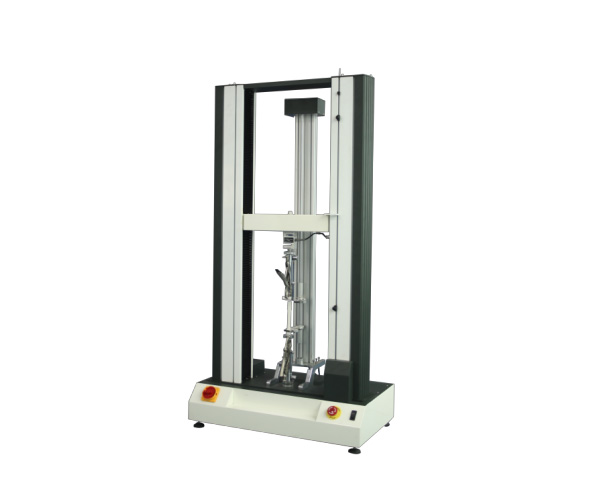Tensile testing machine

b. The moving speed of the movable gripper of the tensile machine must be uniform, which should be (100±20) mm/min. The clamping surface of the gripper should have a serrated surface to ensure that the sample does not move during the stretching process.

c. The minimum length of the clamping surface of the clamp in the direction of the force is 45mm, and the specimen is fixed mechanically or pneumatically. The material and design of the inner surface of the fixture should be such that when the specimen reaches the maximum load, the sliding distance between the specimen and the fixture does not exceed 1% of the initial distance from the fixture.

## (2) Preparation of samples

Cut 6 specimens from the grain surface of the sample block with a die knife. The long sides of the 3 specimens are parallel to the backbone line, and the long sides of the 3 specimens are perpendicular to the backbone line. If the previous test shows that there is sliding between the specimen and the gripper, use a large die cutter. The shape of the cut sample is shown as follows: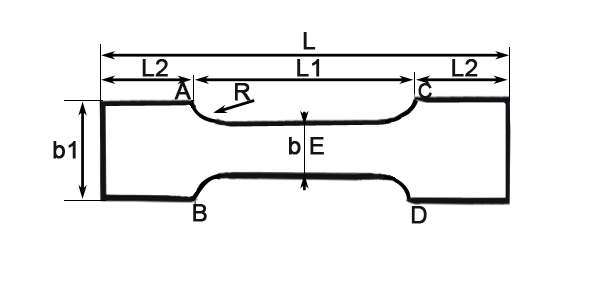The size of the sample is shown in the table below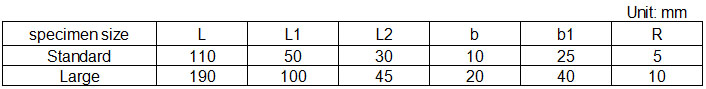The tensile strength is generally measured using standard specimens. If large specimens are used, it should be stated in the report.

After cutting the sample according to the above method, put the sample in standard air for air conditioning.

## (3) Measuring method

① Measurement of the cross-sectional area of the sample: Determine the thickness of the three points a, b, and c of the sample as shown in the figure below according to the thickness measurement regulations, and use the arithmetic average of these three points as the thickness of the sample. Then use a vernier caliper to measure the width of the three points a, b, and c of the sample’s grain surface and meat surface to the accuracy of 0.1mm, and the arithmetic average of the six measured values is used as the width of the sample. The widths of the three points a, b, and c are calculated using the average of the width of the grain and meat respectively. Then:

The cross-sectional area of the sample = the width of the sample × the thickness of the sample

Cross-sectional area of point a = average width of point a × thickness of point a
Cross-sectional area of point b = average width of point b × thickness of point b
Cross-sectional area of point c = average width of point c × thickness of point c

Note: For soft leather, it is inconvenient to measure the average width, and the width of the die cutter can be used as the width of the sample.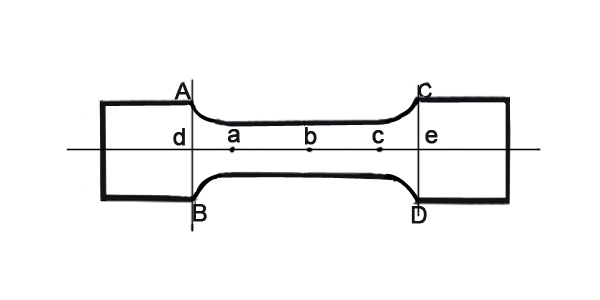② Determination of tensile strength

a.Adjust the weight of the tensile machine so that the maximum load does not exceed 5 times (estimated) of the force that the specimen can withstand, and adjust the moving speed of the movable clamp of the tensile machine to (100±20) mm/min.

b.Correct the pointer on the reading plate of the tensile tester to “0”, (the electronic tensile tester is directly adjusted to 0).

c.Adjust the distance between the upper and lower clamps of the tensile machine to 50mm (for standard specimen) or 100mm (for large specimen), clamp the specimen in the upper and lower clamps, and align the AB line and CD line of the specimen with the edges of the clamps. After clamping the sample, its grain surface should be on a flat surface without distortion.

d. Start the machine until the sample breaks, and record the maximum force value at the break.

## (4) Calculation

The tensile strength σ (MPa) of the sample is calculated according to the following formula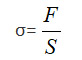where
σ–tensile strength, MPa;
F–Maximum force value when the sample breaks, N;
S–The cross-sectional area of the sample break point, mm².

Calculate the average value of the tensile strength of all samples, and the calculation result is expressed as the average value.

## (5) Attention

① If the break point is at any point of a, b, c, the cross-sectional area of the point is used to calculate the result; if the break point is between two points, the average of the cross-sectional areas of the two points is used to calculate the result.

② Air conditioning must be carried out before measuring the tensile strength, otherwise the results will vary greatly. Because the moisture in the leather has a great influence on the tensile strength, for example, If the back of the air-dried bottom leather is soaked to 50% humidity, the tensile strength can be increased to 1.55 times the original value.

③If the sample slips out of the holder during the stress process, this data should be discarded, and another sample should be retested.

Amadetech is a professional Leather testing instruments supplier from China, offering various lab testing equipment with decent quality in competitive price. Please click Universal Tensile Testing Machine to learn more, or directly reach us by [email protected].